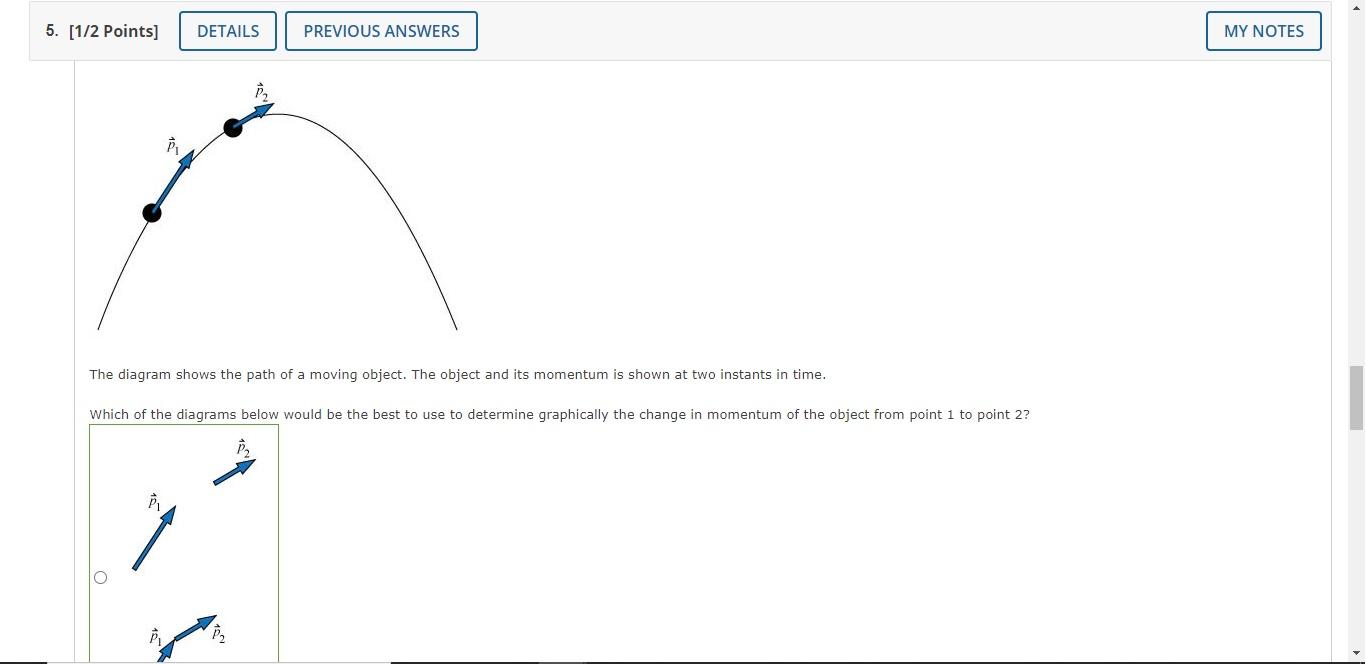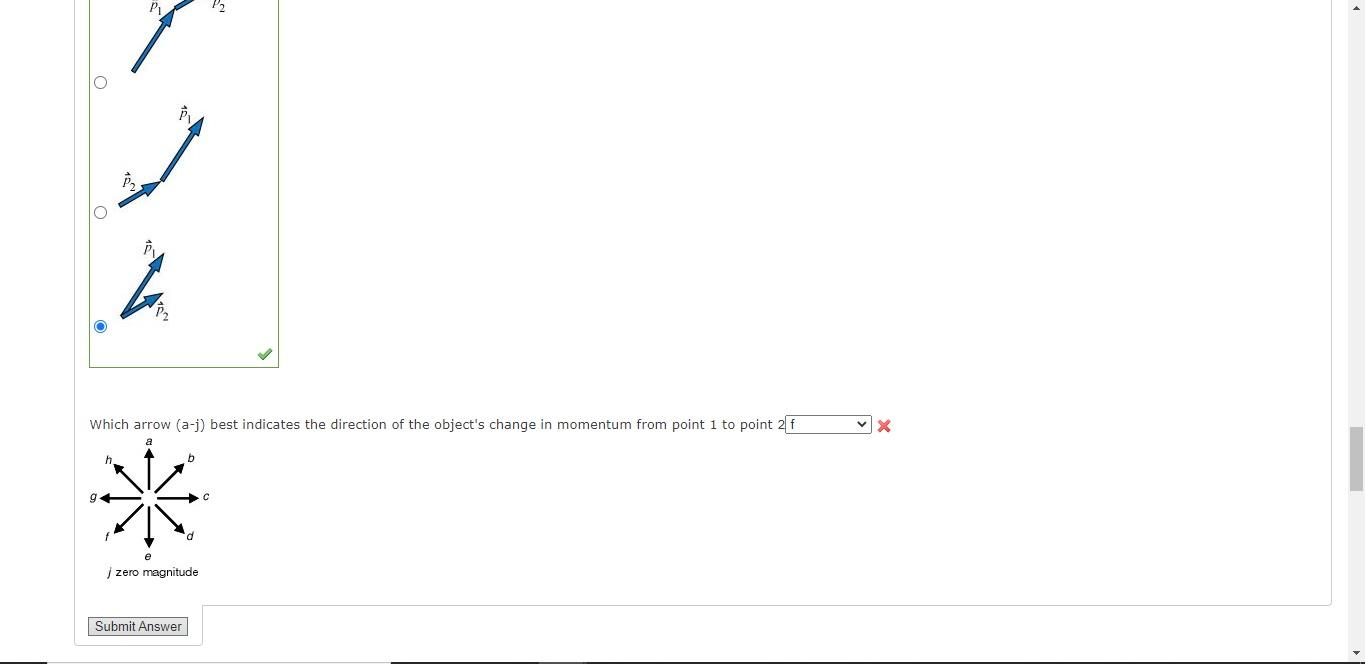# Question Solved1 AnswerWhich arrow (a-j) best indicates the direction of the object's change in momentum from point 1 to point 2? 5. [1/2 points] DETAILS PREVIOUS ANSWERS MY NOTES The diagram shows the path of a moving object. The object and its momentum is shown at two instants in time. Which of the diagrams below would be the best to use to determine graphically the change in momentum of the object from point i to point 2? P P2 P Which arrow (a-j) best indicates the direction of the object's change in momentum from point 1 to point 27 x g * d e i zero magnitude Submit AnswerWhich arrow (a-j) best indicates the direction of the object's change in momentum from point 1 to point 2?

Transcribed Image Text: 5. [1/2 points] DETAILS PREVIOUS ANSWERS MY NOTES The diagram shows the path of a moving object. The object and its momentum is shown at two instants in time. Which of the diagrams below would be the best to use to determine graphically the change in momentum of the object from point i to point 2? P P2 P Which arrow (a-j) best indicates the direction of the object's change in momentum from point 1 to point 27 x g * d e i zero magnitude Submit Answer
More
Transcribed Image Text: 5. [1/2 points] DETAILS PREVIOUS ANSWERS MY NOTES The diagram shows the path of a moving object. The object and its momentum is shown at two instants in time. Which of the diagrams below would be the best to use to determine graphically the change in momentum of the object from point i to point 2? P P2 P Which arrow (a-j) best indicates the direction of the object's change in momentum from point 1 to point 27 x g * d e i zero magnitude Submit Answer